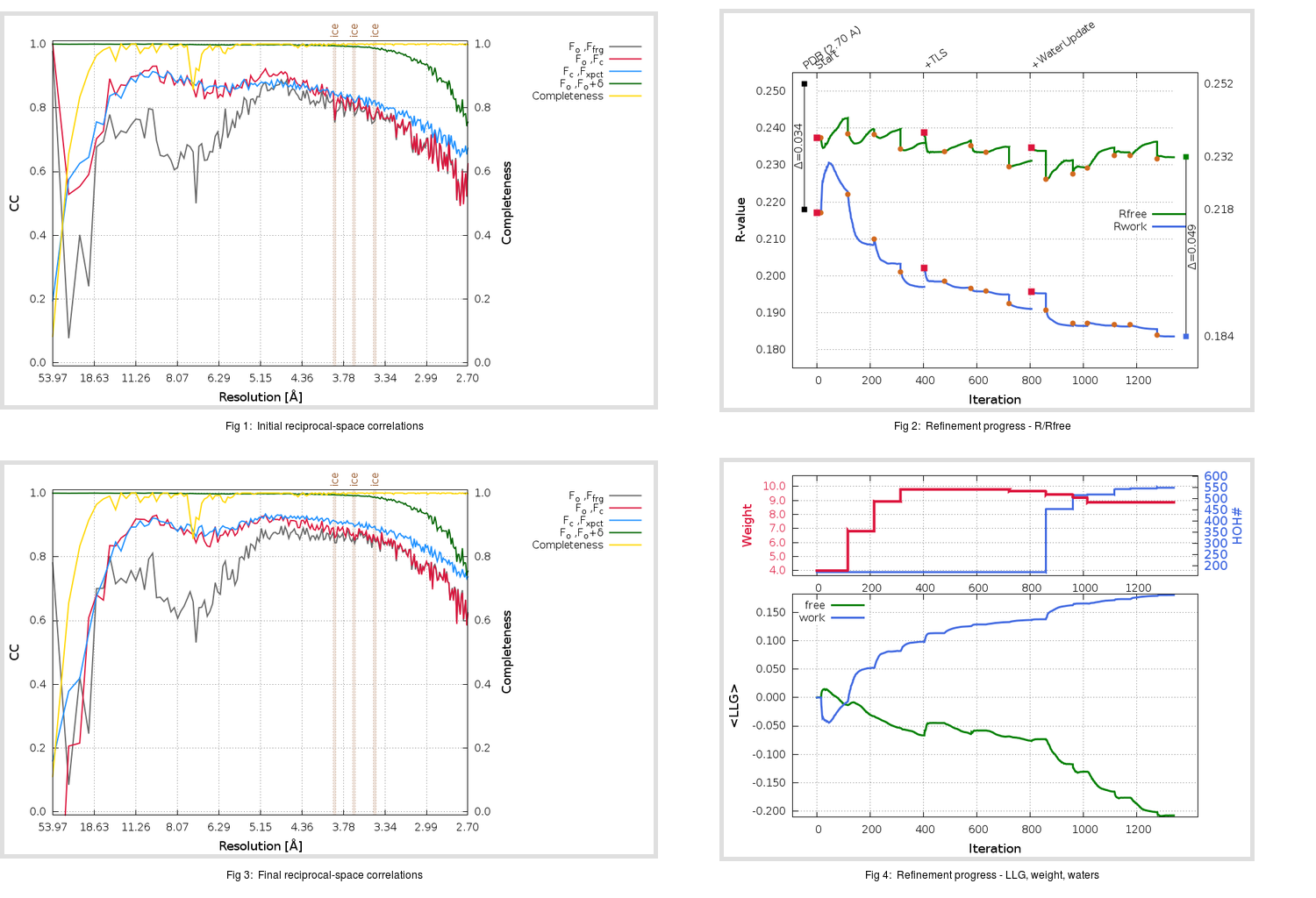Content:

```    Diffraction limits & principal axes of ellipsoid fitted to diffraction cut-off surface:
2.979         1.0000   0.0000   0.0000       a*
3.042         0.0000   1.0000   0.0000       b*
2.755         0.0000   0.0000   1.0000       c*
```

## Deposited

` `
 Date deposited Date data collection Resolution R, Rfree 20090512 20071207 2.70 0.2170 0.2520

Molprobity (CCP4 7.0 version) summary:

```Ramachandran outliers =   0.23 %
favored =  96.66 %
Rotamer outliers      =   1.46 %
C-beta deviations     =     0
Clashscore            =   9.04
RMS(bonds)            =   0.0025
RMS(angles)           =   0.57
MolProbity score      =   1.82
Resolution            =   2.70
R-work                =   0.2170
R-free                =   0.2520
```

```Number of waters      =   172

<B> (all atoms) =   68.22 ( sd =   30.03 ) for      14442 non-hydrogen atoms
<B>   (protein) =   67.95 ( sd =   29.74 ) for      14081 non-hydrogen atoms
<B>     (water) =   48.18 ( sd =   11.36 ) for        172 non-hydrogen atoms
<B>    (others) =  106.65 ( sd =   32.56 ) for        189 non-hydrogen atoms

B min/max       (all non-hydrogen atoms) =   23.42 /  558.88
B min/max   (protein non-hydrogen atoms) =   23.42 /  558.88
B min/max     (water non-hydrogen atoms) =   26.05 /   92.88
B min/max     (other non-hydrogen atoms) =   44.40 /  166.57
```

## BUSTER (re-)refinement

` `

Molprobity (CCP4 7.0 version) summary:

```Ramachandran outliers =   0.57 %
favored =  95.82 %
Rotamer outliers      =   8.85 %
C-beta deviations     =     7
Clashscore            =   5.10
RMS(bonds)            =   0.0121
RMS(angles)           =   1.63
MolProbity score      =   2.28
Resolution            =   2.70
R-work                =   0.1837
R-free                =   0.2322
```

```Number of waters      =   549

<B> (all atoms) =   59.33 ( sd =   22.13 ) for      14819 non-hydrogen atoms
<B>   (protein) =   59.27 ( sd =   22.26 ) for      14081 non-hydrogen atoms
<B>     (water) =   52.07 ( sd =   11.20 ) for        549 non-hydrogen atoms
<B>    (others) =   84.85 ( sd =   17.75 ) for        189 non-hydrogen atoms

B min/max       (all non-hydrogen atoms) =   20.60 /  215.49
B min/max   (protein non-hydrogen atoms) =   27.44 /  215.49
B min/max     (water non-hydrogen atoms) =   20.60 /  122.71
B min/max     (other non-hydrogen atoms) =   51.48 /  113.84
```

Refinement progression:Results:

` `
 File Remark 2WII_aB_refine.01_03_refine.pdb.gz exact refinement commands are in header 2WII_aB_refine.01_03_refine.mtz.gz including original deposited data and several re-refinement map coefficients 2WII_aB_refine.01_03_BUSTER_model.cif.gz including any non-standard compound restraints 2WII_aB_refine.01_03_BUSTER_refln.cif.gz